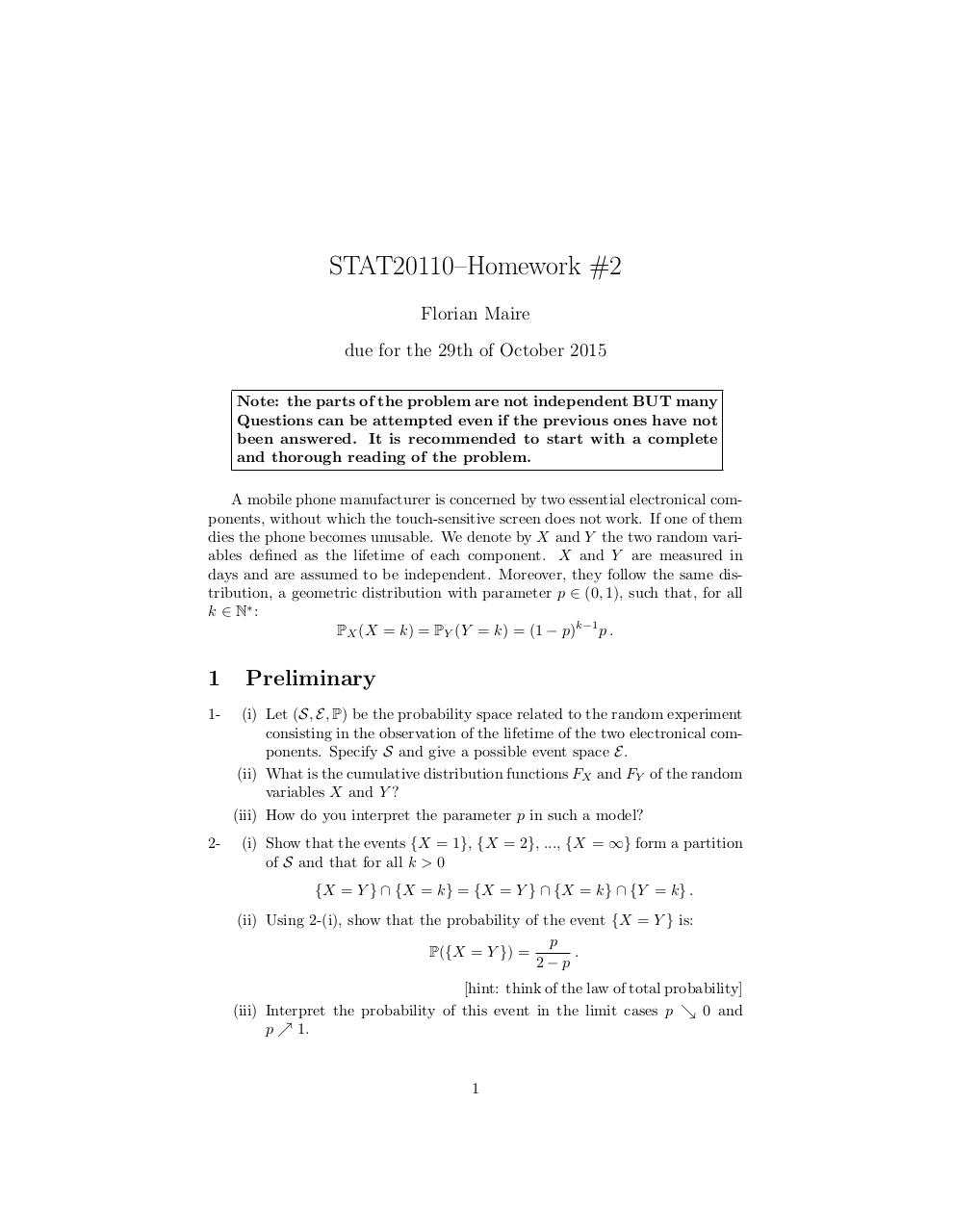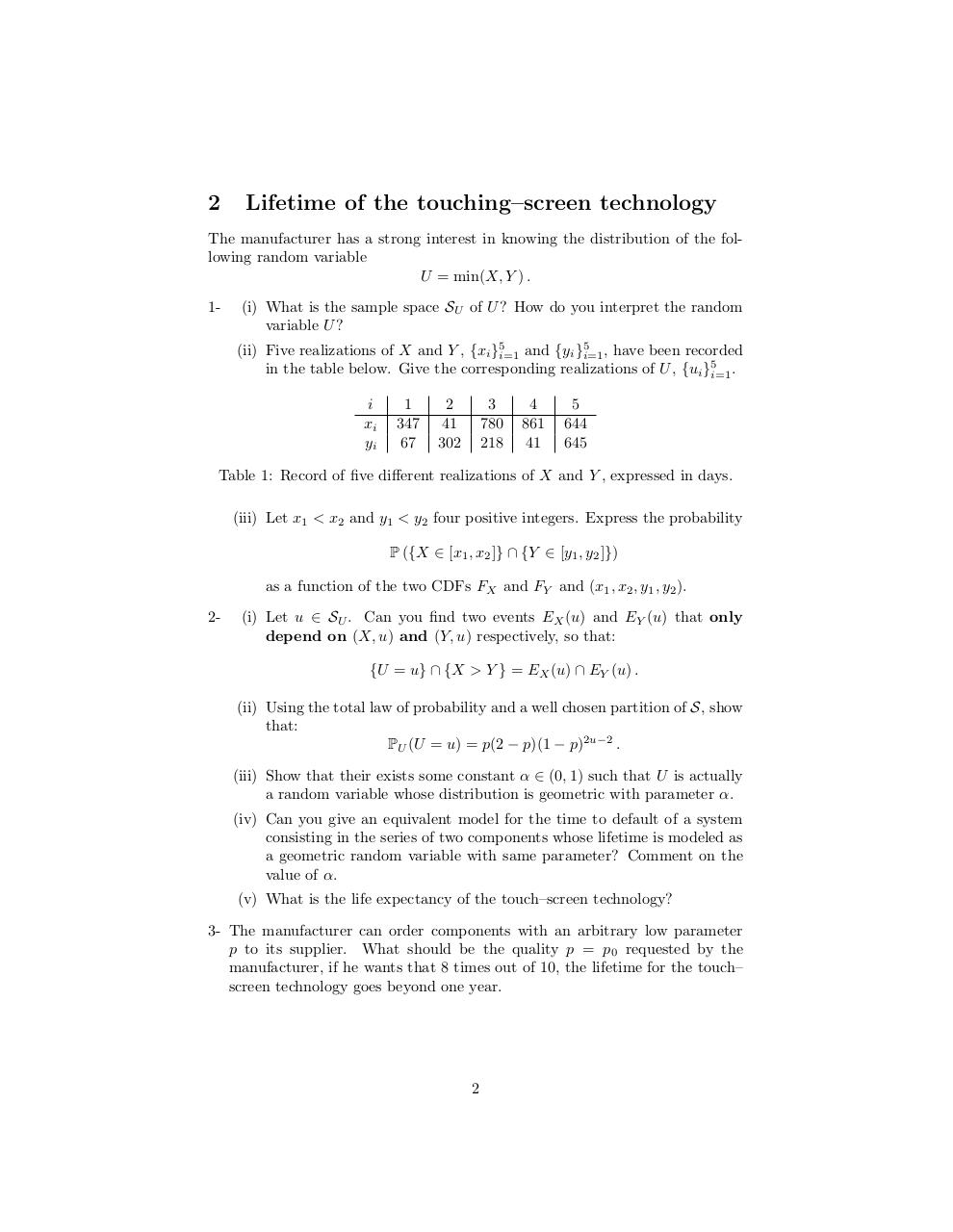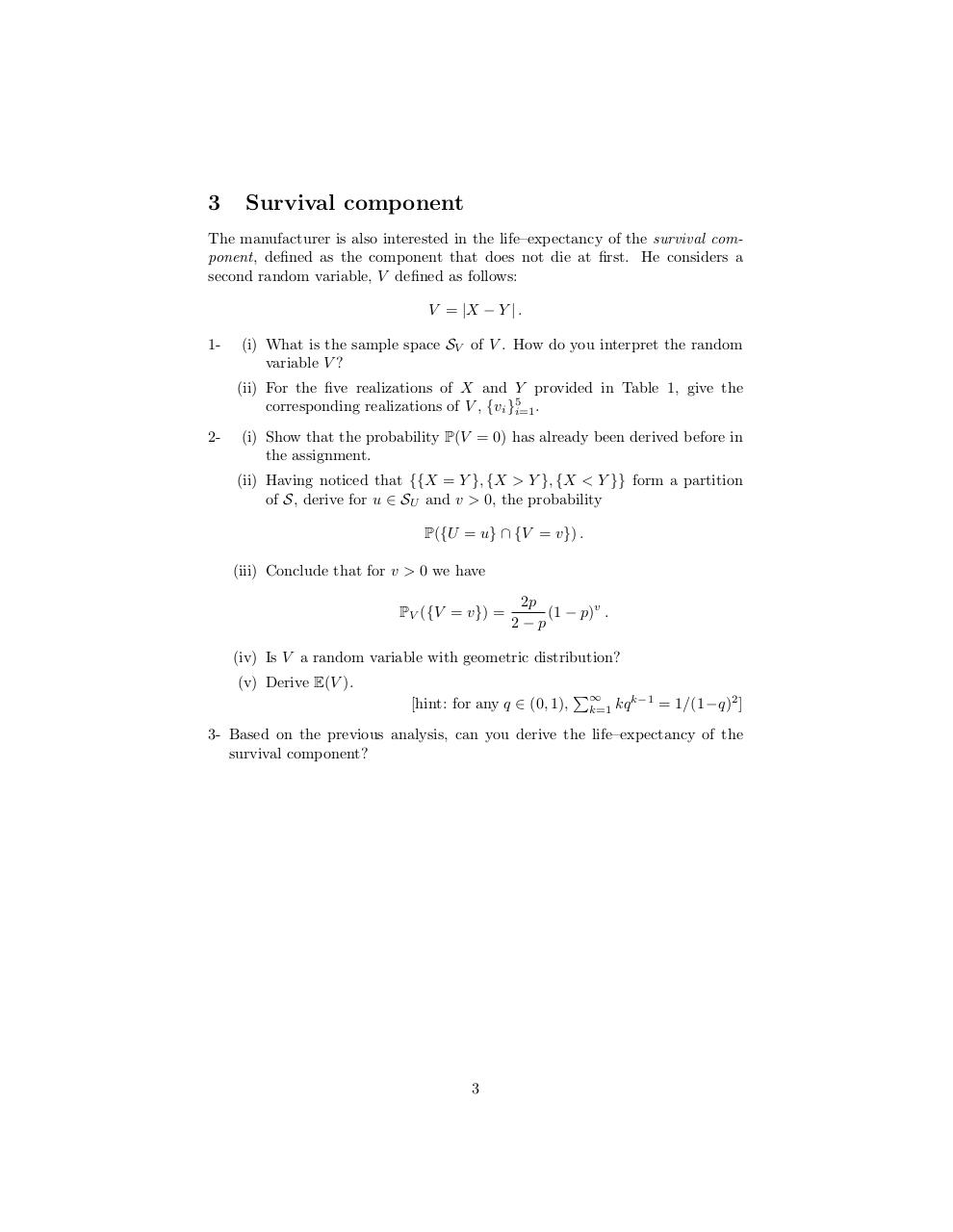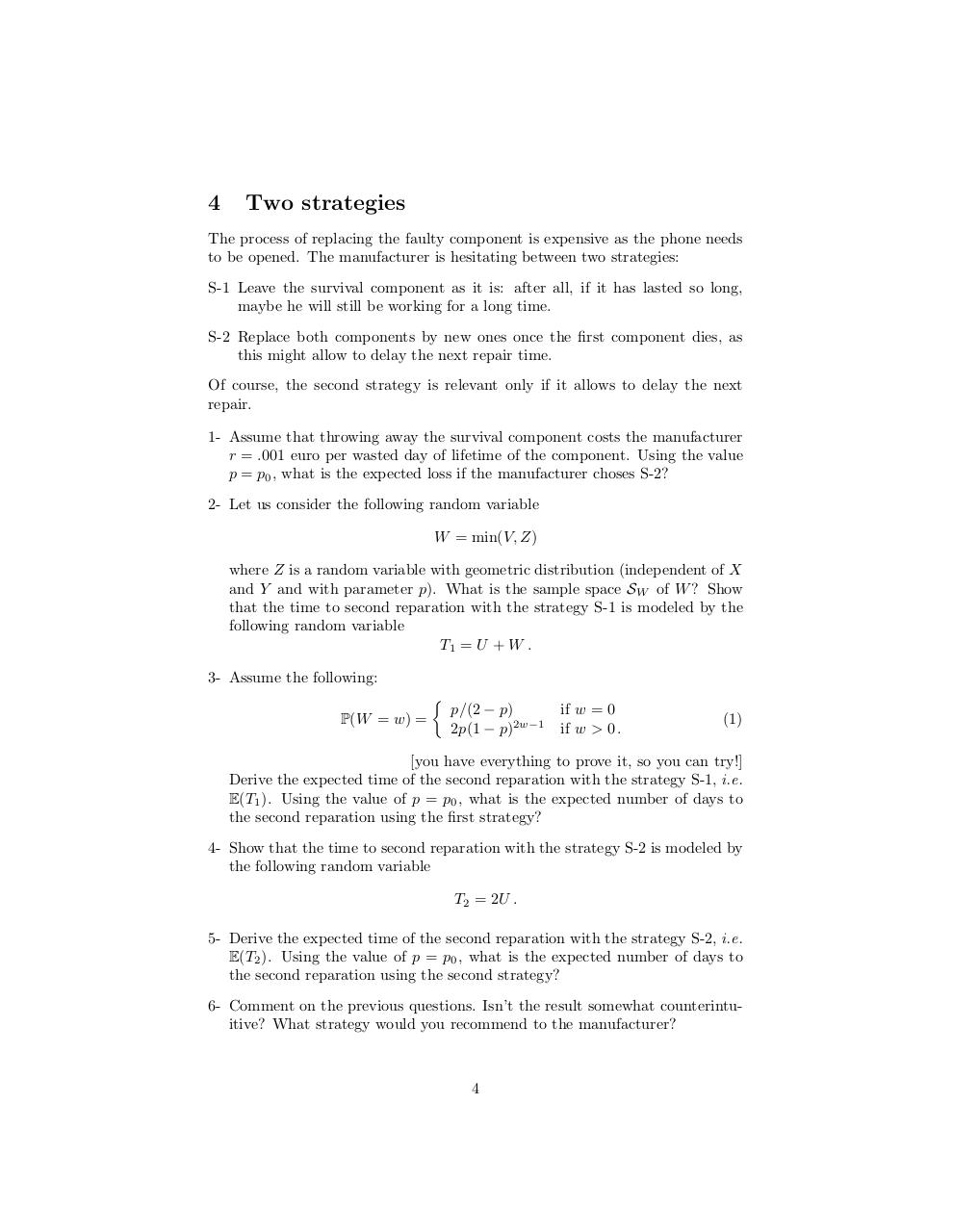# Probability Theory .pdf

### File information

Original filename: Probability Theory.pdf

This PDF 1.5 document has been generated by TeX / MiKTeX pdfTeX-1.40.14, and has been sent on pdf-archive.com on 19/10/2015 at 20:16, from IP address 109.77.x.x. The current document download page has been viewed 575 times.
File size: 155 KB (4 pages).
Privacy: public file

Probability Theory.pdf (PDF, 155 KB)

### Document preview

STAT20110–Homework #2
Florian Maire
due for the 29th of October 2015
Note: the parts of the problem are not independent BUT many
Questions can be attempted even if the previous ones have not
been answered. It is recommended to start with a complete
and thorough reading of the problem.
A mobile phone manufacturer is concerned by two essential electronical components, without which the touch-sensitive screen does not work. If one of them
dies the phone becomes unusable. We denote by X and Y the two random variables defined as the lifetime of each component. X and Y are measured in
days and are assumed to be independent. Moreover, they follow the same distribution, a geometric distribution with parameter p ∈ (0, 1), such that, for all
k ∈ N∗ :
PX (X = k) = PY (Y = k) = (1 − p)k−1 p .

1

Preliminary

1-

(i) Let (S, E, P) be the probability space related to the random experiment
consisting in the observation of the lifetime of the two electronical components. Specify S and give a possible event space E.
(ii) What is the cumulative distribution functions FX and FY of the random
variables X and Y ?
(iii) How do you interpret the parameter p in such a model?

2-

(i) Show that the events {X = 1}, {X = 2}, ..., {X = ∞} form a partition
of S and that for all k &gt; 0
{X = Y } ∩ {X = k} = {X = Y } ∩ {X = k} ∩ {Y = k} .
(ii) Using 2-(i), show that the probability of the event {X = Y } is:
p
P({X = Y }) =
.
2−p
[hint: think of the law of total probability]
(iii) Interpret the probability of this event in the limit cases p &amp; 0 and
p % 1.

1

2

Lifetime of the touching–screen technology

The manufacturer has a strong interest in knowing the distribution of the following random variable
U = min(X, Y ) .
1-

(i) What is the sample space SU of U ? How do you interpret the random
variable U ?
(ii) Five realizations of X and Y , {xi }5i=1 and {yi }5i=1 , have been recorded
in the table below. Give the corresponding realizations of U , {ui }5i=1 .
i
xi
yi

1
347
67

2
41
302

3
780
218

4
861
41

5
644
645

Table 1: Record of five different realizations of X and Y , expressed in days.
(iii) Let x1 &lt; x2 and y1 &lt; y2 four positive integers. Express the probability
P ({X ∈ [x1 , x2 ]} ∩ {Y ∈ [y1 , y2 ]})
as a function of the two CDFs FX and FY and (x1 , x2 , y1 , y2 ).
2-

(i) Let u ∈ SU . Can you find two events EX (u) and EY (u) that only
depend on (X, u) and (Y, u) respectively, so that:
{U = u} ∩ {X &gt; Y } = EX (u) ∩ EY (u) .
(ii) Using the total law of probability and a well chosen partition of S, show
that:
PU (U = u) = p(2 − p)(1 − p)2u−2 .
(iii) Show that their exists some constant α ∈ (0, 1) such that U is actually
a random variable whose distribution is geometric with parameter α.
(iv) Can you give an equivalent model for the time to default of a system
consisting in the series of two components whose lifetime is modeled as
a geometric random variable with same parameter? Comment on the
value of α.
(v) What is the life expectancy of the touch–screen technology?

3- The manufacturer can order components with an arbitrary low parameter
p to its supplier. What should be the quality p = p0 requested by the
manufacturer, if he wants that 8 times out of 10, the lifetime for the touch–
screen technology goes beyond one year.

2

3

Survival component

The manufacturer is also interested in the life–expectancy of the survival component, defined as the component that does not die at first. He considers a
second random variable, V defined as follows:
V = |X − Y | .
1-

(i) What is the sample space SV of V . How do you interpret the random
variable V ?
(ii) For the five realizations of X and Y provided in Table 1, give the
corresponding realizations of V , {vi }5i=1 .

2-

(i) Show that the probability P(V = 0) has already been derived before in
the assignment.
(ii) Having noticed that {{X = Y }, {X &gt; Y }, {X &lt; Y }} form a partition
of S, derive for u ∈ SU and v &gt; 0, the probability
P({U = u} ∩ {V = v}) .
(iii) Conclude that for v &gt; 0 we have
PV ({V = v}) =

2p
(1 − p)v .
2−p

(iv) Is V a random variable with geometric distribution?
(v) Derive E(V ).
[hint: for any q ∈ (0, 1),

P∞

k=1

kq k−1 = 1/(1−q)2 ]

3- Based on the previous analysis, can you derive the life–expectancy of the
survival component?

3

4

Two strategies

The process of replacing the faulty component is expensive as the phone needs
to be opened. The manufacturer is hesitating between two strategies:
S-1 Leave the survival component as it is: after all, if it has lasted so long,
maybe he will still be working for a long time.
S-2 Replace both components by new ones once the first component dies, as
this might allow to delay the next repair time.
Of course, the second strategy is relevant only if it allows to delay the next
repair.
1- Assume that throwing away the survival component costs the manufacturer
r = .001 euro per wasted day of lifetime of the component. Using the value
p = p0 , what is the expected loss if the manufacturer choses S-2?
2- Let us consider the following random variable
W = min(V, Z)
where Z is a random variable with geometric distribution (independent of X
and Y and with parameter p). What is the sample space SW of W ? Show
that the time to second reparation with the strategy S-1 is modeled by the
following random variable
T1 = U + W .
3- Assume the following:

P(W = w) =

p/(2 − p)
2p(1 − p)2w−1

if w = 0
if w &gt; 0 .

(1)

[you have everything to prove it, so you can try!]
Derive the expected time of the second reparation with the strategy S-1, i.e.
E(T1 ). Using the value of p = p0 , what is the expected number of days to
the second reparation using the first strategy?
4- Show that the time to second reparation with the strategy S-2 is modeled by
the following random variable
T2 = 2U .
5- Derive the expected time of the second reparation with the strategy S-2, i.e.
E(T2 ). Using the value of p = p0 , what is the expected number of days to
the second reparation using the second strategy?
6- Comment on the previous questions. Isn’t the result somewhat counterintuitive? What strategy would you recommend to the manufacturer?

4Use the permanent link to the download page to share your document on Facebook, Twitter, LinkedIn, or directly with a contact by e-Mail, Messenger, Whatsapp, Line..

Use the short link to share your document on Twitter or by text message (SMS)

#### HTML Code

Copy the following HTML code to share your document on a Website or Blog

#### QR Code### Related keywords